# Class 11 Maths NCERT Solutions for Chapter 9 Sequences and Series Miscellaneous Exercise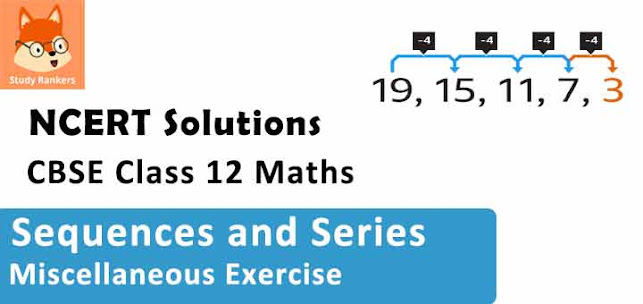### Sequences and Series Miscellaneous Exercise Solutions

1. Show that the sum of (m + n)th and (m – n)th terms of an A.P. is equal to twice the mth term.

Solution

Let a and d be the first term and the common difference of the A.P. respectively.
It is known that the kth term of an A. P. is given by
ak = a + (k –1) d
∴ am+n = a + (m + n –1) d
amn = a + (m – n –1) d
a= a + (m –1) d
∴ am+n + am-n = a + (m + n –1) d + a + (m – n –1) d
= 2a + (m + n –1 + m – n –1) d
= 2a + (2m – 2) d
= 2a + 2 (m – 1) d
= 2[a + (m – 1) d]
= 2am
Thus, the sum of (m + n)th and (m – n)th terms of an A.P. is equal to twice the mth term.

2. If the sum of three numbers in A.P., is 24 and their product is 440, find the numbers.

Solution

Let the three numbers in A.P. be a – d, a, and a + d.
According to the given information,
(a – d) + (a) + (a + d) = 24 …(1)
⇒ 3a = 24
∴ a = 8
(a – d) a (a + d) = 440 …(2)
⇒ (8 – d) (8) (8 + d) = 440
⇒ (8 – d) (8 + d) = 55
⇒ 64 – d2 = 55
⇒ d2 = 64 – 55 = 9
⇒ d = ± 3
Therefore, when d = 3, the numbers are 5, 8, and 11 and when d = –3, the numbers are 11, 8, and 5.
Thus, the three numbers are 5, 8, and 11.

3. Let the sum of n, 2n, 3n terms of an A.P. be S1, S2 and S3,respectively, show that S3 = 3 (S2– S1).

Solution

Let a and b be the first term and the common difference of the A.P. respectively.
Therefore,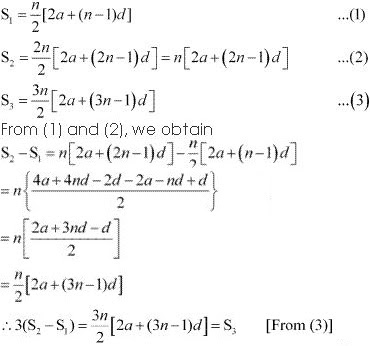Hence, the given result is proved.

4. Find the sum of all numbers between 200 and 400 which are divisible by 7.

Solution

The numbers lying between 200 and 400, which are divisible by 7, are
203, 210, 217, ­­­­­­­­… 399
∴First term, a = 203
Last term, l = 399
Common difference, d = 7
Let the number of terms of the A.P. be n.
∴ an = 399 = a + (n –1) d
⇒ 399 = 203 + (n –1) 7
⇒ 7 (n –1) = 196
⇒ n –1 = 28
⇒ n = 29
∴ S29 = 29/2 (203 + 399)
= (29/2) × 602
= 29 × 301
= 8729
Thus, the required sum is 8729.

5. Find the sum of integers from 1 to 100 that are divisible by 2 or 5.

Solution

The integers from 1 to 100, which are divisible by 2, are 2, 4, 6… 100.
This forms an A.P. with both the first term and common difference equal to 2.
⇒100 = 2 + (n –1) 2
⇒ n = 50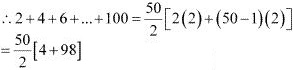= 25 × 102
= 2550
The integers from 1 to 100, which are divisible by 5, are 5, 10… 100.
This forms an A.P. with both the first term and common difference equal to 5.
∴100 = 5 + (n –1) 5
⇒ 5n = 100
⇒ n = 20
∴ 5 + 10 + .... + 100 = 20/2 [2(5) + (20 -1)5]
= 10[10 + 19× 5]
= 10[10 + 95] = 10 × 105
= 1050
The integers, which are divisible by both 2 and 5, are 10, 20, … 100.
This also forms an A.P. with both the first term and common difference equal to 10.
∴100 = 10 + (n –1) (10)
⇒ 100 = 10n
⇒ n = 10
∴ 10 + 20 + ... + 100 = 10/2 [2(10) + (10 - 1)(10)]
= 5[20 + 90] = 5(110) = 550
∴ Required sum  = 2550 + 1050 - 550 = 3050
Thus, the sum of the integers from 1 to 100, which are divisible by 2 or 5, is 3050.

6. Find the sum of all two digit numbers which when divided by 4, yields 1 as remainder.

Solution

The two-digit numbers, which when divided by 4, yield 1 as remainder, are
13, 17, … 97.
This series forms an A.P. with first term 13 and common difference 4.
Let n be the number of terms of the A.P.
It is known that the nth term of an A.P. is given by, an = a + (n –1) d
∴97 = 13 + (n –1) (4)
⇒ 4 (n –1) = 84
⇒ n – 1 = 21
⇒ n = 22
Sum of n terms of an A.P. is given by,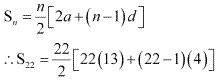= 11[26 + 84]
= 11 × 110
= 1210
Thus, the required sum is  1210.

7. If f is a function satisfying f (x +y) = f(x) f(y) for all x, y ∈ N such that f(1) = 3 and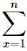f(x) = 120, find the value of n.

Solution

It is given that,
f (x + y) = f (x) × f (y) for all x, y ∈ N …(1)
f (1) = 3
Taking x = y = 1 in (1), we obtain
f (1 + 1) = f (2) = f (1) f (1) = 3 × 3 = 9
Similarly,
f (1 + 1 + 1) = f (3) = f (1 + 2) = f (1) f (2) = 3 × 9 = 27
f (4) = f (1 + 3) = f (1) f (3) = 3 × 27 = 81
∴ f (1), f (2), f (3), …, that is 3, 9, 27, …, forms a G.P. with both the first term and common ratio equal to 3.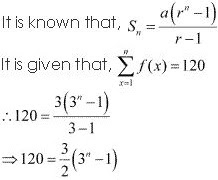⇒ 3n - 1 = 80
⇒ 3n = 81 = 34
∴ n = 4
Thus, the value of n is  4.

8. The sum of some terms of G.P. is 315 whose first term and the common ratio are 5 and 2, respectively. Find the last term and the number of terms.

Solution

Let the sum of n terms of the G.P. be 315.
It is known that,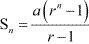It is given that the first term a is 5 and common ratio r is 2.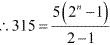⇒ 2n - 1 = 63
⇒ 2n = 64 = 26
⇒ n = 6
Last term of the G.P = 6th  term = ar6–1 = (5)(2)5 = (5)(32) = 160
Thus, the last term of the G.P. is 160.

9. The first term of a G.P. is 1. The sum of the third term and fifth term is 90. Find the common ratio of G.P.

Solution

Let a and r be the first term and the common ratio of the G.P. respectively.
∴ a = 1
a3 = ar2 = r2
a5 = ar4 = r4
∴ r2 + r4 = 90
⇒ r4 + r2 – 90 = 0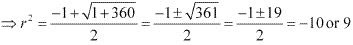∴ r = ± 3 (Taking real roots)
Thus, the common ratio of the G.P. is  ±3.

10. The sum of three numbers in G.P. is 56. If we subtract 1, 7, 21 from these numbers in that order, we obtain an arithmetic progression. Find the numbers.

Solution

Let the three numbers in G.P. be aar, and ar2.
From the given condition, a + ar + ar2 = 56
⇒ a (1 + r + r2) = 56
⇒ a = 56/(1 + r + r2 )  ...(1)
a – 1, ar – 7, ar2 – 21 forms an A.P.
∴(ar – 7) – (a – 1) = (ar2 – 21) – (ar – 7)
⇒ ar – a – 6 = ar– ar – 14
⇒ar– 2ar + a = 8
⇒ar– ar – ar + a = 8
⇒a(r+ 1 – 2r) = 8
⇒ a (r – 1)2 = 8 …(2)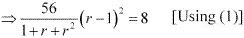⇒7(r2 – 2r + 1) = 1 + r + r2
⇒7r2 – 14 r + 7 – 1 – r – r2 = 0
⇒ 6r2 – 15r + 6 = 0
⇒ 6r2 – 12r – 3r + 6 = 0
⇒ 6r (r – 2) – 3 (r – 2) = 0
⇒ (6r – 3) (r – 2) = 0
∴ r = 2, 1/2
When r = 2, a = 8
When r = 1/2, a = 32
Therefore, when r = 2, the three numbers in G.P. are  8, 16 and 32.
When r = 1/2, the three numbers in G.P. are  32, 16 and 8.
Thus, in either case, the three required numbers are 8, 16, and 32.

11. A G.P. consists of an even number of terms. If the sum of all the terms is 5 times the sum of terms occupying odd places, then find its common ratio.

Solution

Let the G.P. be T1, T2, T3, T4, … T2n.
Number of terms = 2n
According to the given condition,
T1 + T2 + T3 + …+ T2n = 5 [T1 + T3 + … +T2n–1]
⇒ T1 + T2 + T3 + … + T2n – 5 [T1 + T3 + … + T2n–1] = 0
⇒ T2 + T4 + … + T2n = 4 [T1 + T3 + … + T2n–1]
Let the G.P. be aarar2ar3, …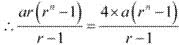⇒  ar = 4a
⇒  r = 4
Thus, the common ratio of the G.P. is 4.

12. The sum of the first four terms of an A.P. is 56. The sum of the last four terms is 112. If its first term is 11, then find the number of terms.

Solution

Let the A.P. be aa + da + 2da + 3d, ... a + (n – 2) da + (n – 1)d.
Sum of first four terms = a + (a + d) + (a + 2d) + (a + 3d) = 4a + 6d
Sum of last four terms = [a + (n – 4) d] + [a + (n – 3) d] + [a + (n – 2) d] + [a + n – 1) d]
= 4a + (4n – 10) d
According to the given condition,
4a + 6d = 56
⇒ 4(11) + 6d = 56 [Since a = 11 (given)]
⇒ 6d = 12
⇒ d = 2
∴ 4a + (4n –10) d = 112
⇒ 4(11) + (4n – 10)2 = 112
⇒ (4n – 10)2 = 68
⇒ 4n – 10 = 34
⇒ 4n = 44
⇒ n = 11
Thus, the number of terms of the A.P. is 11.

13. If (a + bx)/(a - bx) = (b + cx)/(b - cx) = (c + dx)(c - dx)    (x ≠ 0) then show that a, b, c and d are in G.P.

Solution

It is given that,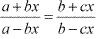⇒ (a + bx)(b - cx) = (b + cx)(a - bx)
⇒ ab - acx + b2 x - bcx2 = ab - b2 x + acx - bcx2
⇒ 2b2 x = 2acx
⇒ b2 = ac
⇒ b/a = c/b  ...(1)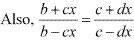⇒ (b + cx)(c - dx) = (b - cx)(c + dx)
⇒ bc - bdx + c2 x - cdx2 = bc + bdx - c2 x - cdx2
⇒ 2c2 x =2bdx
⇒ c2 = bd
⇒ c/d = d/c ...(2)
From (1) and (2), we obtain
b/a = c/b = d/c
Thus, a, b, c, and d are in G.P.

14. Let S be the sum, P the product and R the sum of reciprocals of n terms in a G.P. Prove that P2R= Sn

Solution

Let the G.P. be a, ar, ar2, ar3, … arn – 1
According to the given information,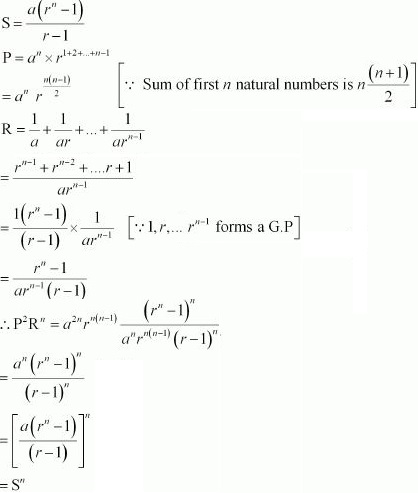Hence, P2 Rn = Sn

15. The pthqth and rth terms of an A.P. are a, b, c respectively. Show that (q – r)a + (r – p)b + (p – q)c = 0

Solution

Let t and d be the first term and the common difference of the A.P. respectively.
The nth term of an A.P. is given by, an= t + (n – 1) d
Therefore,
ap = t + (p – 1) d = a …(1)
aq = t + (q – 1)d = b …(2)
ar = t + (r – 1) d = c …(3)
Subtracting equation (2) from (1), we obtain
(p – 1 – q + 1) d = a – b
⇒ (p – q) d = a – b
∴ d = (a - b)/(p - q)  ...(4)
Subtracting equation (3) from (2), we obtain
(q – 1 – r + 1) d = b – c
⇒ (q – r) d = b – c
⇒ d = (b - c)/(q - r) ...(5)
Equating both the values of d obtained in (4) and (5), we obtain /
(a - b)/(p - q) = (b - c)/(q - r)
⇒ (a - b)(q - r) = (b - c)(p - q)
⇒ aq - bq - ar + br = bp  - bq - cp + cq
⇒ bp - cp  + cq - aq + ar - br = 0
⇒ (-aq + ar) + (bp - br) + (-cp + cq) = 0  (By rearranging terms)
⇒ -a(q - r) - b(r - p)- c(p - q) = 0
⇒ a(q - r) + b(r - p) + c(p - q) = 0
Thus, the given result is proved.

16. If a (1/b + 1/c), b(1/c + 1/a), c(1/a + 1/b) are in A.P., prove that, a, b, c are in A.P.

Solution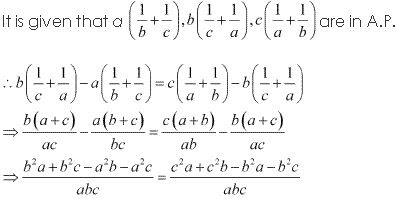⇒ b2a - a2b + b2c - a2c = c2a - b2a + c2b - b2
⇒ ab(b - a) + c(b2 - a2) = a(c2 - b2) + bc(c - b)
⇒ ab(b - a) + c(b - a)(b + a) = a(c - b)(c + b) + bc(c - b)
⇒ (b - a)(ab + cb + ca) = (c - b)(ac + ab + bc)
⇒ b - a = c - b
Thus, a, b, and c are in A.P.

17. If a, b, c, d are in G.P, prove that (an + bn), (bn + cn), (cn + dn) are in G.P.
Solution
Itt is given that a, b, c, and d are in G.P.
∴ b2 = ac …(1)
c2 = bd …(2)
It has to be proved that (an + bn), (bn + cn), (cn + dn) are in G.P. i.e.,
(bn + cn)2 = (an + bn) (cn + dn)
Consider L.H.S.
(bn + cn)2 = b2+ 2bncn + c2n
= (b2)n+ 2bncn + (c2) n
= (ac)n + 2bncn + (bd)n  [Using (1) and (2)]
= an cn + bncn+ bn cn + bn dn
= an cn + bncn+ an dn + bn dn  [Using (3)]
= cn (an + bn) + dn (an + bn)
= (an + bn) (cn + dn)
= R.H.S.
∴ (bn + cn)2 = (an + bn) (cn + dn)
Thus, (an + bn), (bn + cn), and (cn + dn) are in G.P.

18. If a and are the roots of are roots of x2 – 3x + p = 0 , and c, d are roots of x2 – 12x + q = 0, where a, b, cd, form a G.P. Prove that (q + p): (q – p) = 17:15.
Solution

It is given that a and b are the roots of x– 3x + p = 0
∴ a + b = 3 and ab = p …(1)
Also, c and d are the roots of x2 - 12x + q = 0
∴c + d = 12 and cd = q …(2)
It is given that a, b, c, d are in G.P.
Let a = x, b = xr, c = xr2, d = xr3
From (1) and (2), we obtain
x + xr = 3
⇒ x (1 + r) = 3
xr2 + xr3 =12
⇒ xr(1 + r) = 12
On dividing, we obtain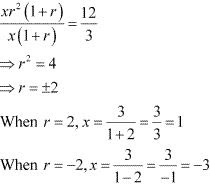Case I :

When r = 2 and x = 1
ab = x2 r = 2
cd = x2 r5 = 32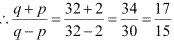i.e., (q + p) : (q - p) = 17 : 15

Case II :
when r = -2, x = -3
ab = x2 r = -18
cd  = x2 r5 = -288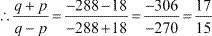i.e., (q + p) : (q - p) = 17 : 15
Thus, in both the cases, we obtain (q + p) : (q - p) = 17 : 15

19. The ratio of the A. M and G.M. of two positive numbers a and b, is m : n . Show that a: b = (m + √(n2 - n2) : (m - √m2 - n2) .
Solution
Let the two numbers be a and b.
A. M  = (a + b)/2 and G.M. = √ab
According to the given condition,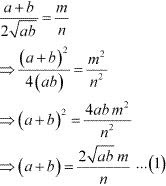Using this in the identity (a - b)2 = (a + b)2 - 4ab, we obtain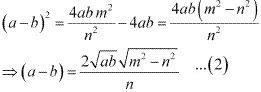Adding (1) and (2), we obtain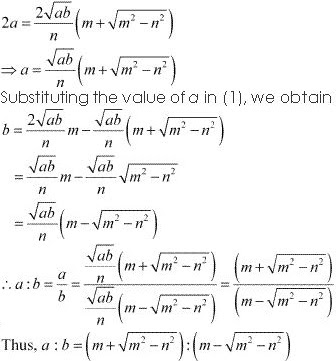20. If a, b, c are in A.P., b, c, d are in G.P and 1/c, 1/d, 1/e are in A.P. prove that a, c, e are in G.P.
Solution

It is given that a, b, c are in A.P.
∴ b – a = c – b …(1)
It is given that b, c, d, are in G.P.
∴ c2 = bd … (2)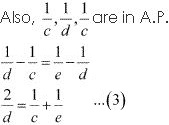It has to be proved that a, c, e are in G.P. i.e., c2 = ae
From (1), we obtain
2b = a + c
⇒ b = (a + c)/2
From(2), we obtain
d = c2 /b
Substituting these values in (3), we obtain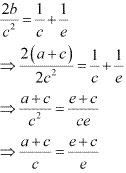⇒ (a + c)e = (e + c)c
⇒ ae + ce = ec + c2
⇒ c2 = ae
Thus, a, c, and e are in G.P.

21. Find the sum of the following series up to n terms:
(i) 5 + 55 + 555 + …
(ii) .6 +.66 +. 666 +…

Solution

(i) 5 + 55 + 555 +…
Let Sn = 5 + 55 + 555 + ... to n terms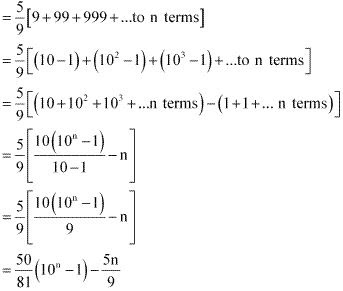(ii) 0.6 + 0.66 + 0.666 + .....
Let Sn = 0.6 + 0.66 +0.666 + .... to n terms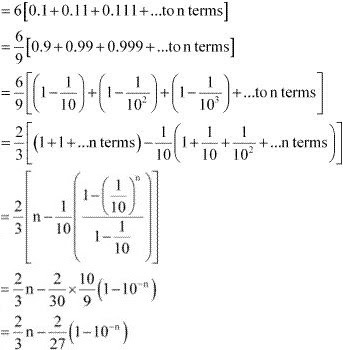22. Find the 20th term of the series 2 × 4 + 4 × 6 + 6 × 8 + … + n terms.

Solution

The given series is 2 × 4 + 4 × 6 + 6 × 8 + … n terms
∴ nth term = an= 2n × (2n + 2) = 4n2 + 4n
a20 = 4 (20)2 + 4(20) = 4 (400) + 80 = 1600 + 80 = 1680
Thus, the 20th term of the series is 1680.

23. Find the sum of the first n terms of the series: 3 + 7 + 13 + 21 + 31 + …

Solution

The given series is 3 + 7 + 13 + 21 + 31 + …
S = 3 + 7 + 13 + 21 + 31 + …+ an–1 an
S = 3 + 7 + 13 + 21 + …. + an – 2 a– 1 + an
On subtracting both the equations, we obtain
S – S = [3 + (7 + 13 + 21 + 31 + …+ an–1 an)] – [(3 + 7 + 13 + 21 + 31 + …+ an–1)+ an]
S – S = 3 + [(7 – 3) + (13 – 7) + (21 – 13) + … + (an – an–1)]– an
0 = 3 + [4 + 6 + 8 + … (n –1) terms] – an
an = 3 + [4 + 6 + 8 + … (n –1) terms]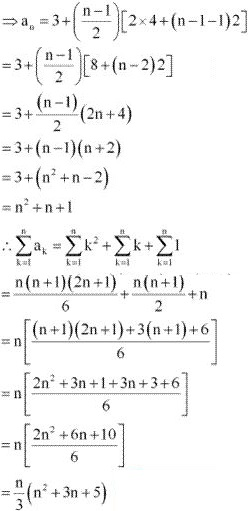24. If S1, S2, S3 are the sum of first n natural numbers, their squares and their cubes, respectively, show that 9S22 =S3 (1 + 8S1)

Solution

From the given information,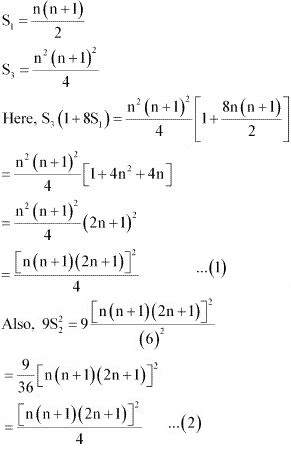Thus, from (1) and (2), we obtain 9S22 =S3 (1 + 8S1)

25. Find the sum of the following series up to n terms  13/1 + (13 + 23)/(1 + 3) + (13 + 23 + 33)/(1 + 3 + 5)  + ....

Solution

The nth term of the given series is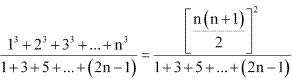Here, 1, 3, 5, ... (2n - 1) is an A.P. with first term a, last term (2n - 1) and number of terms as n
∴ 1 + 3 + 5 +  ... + (2n - 1) = n/2 [2 × 1 + (n - 1)2] = n2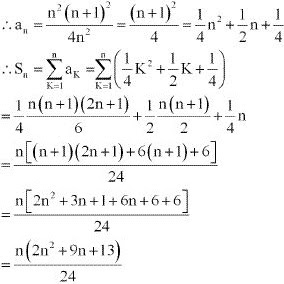26. Show that [1 × 22 + 2 × 32 + .... + n × (n + 1)2]/[12 × 2 + 22 × 3 + ... + n2 × (n + 1)] = (3n + 5)/(3n + 1)

Solution

nth term of the numerator  = n(n + 1)2 = n3 + 2n2 + n
nth term of the denominator = n2 (n + 1) = n3 + n2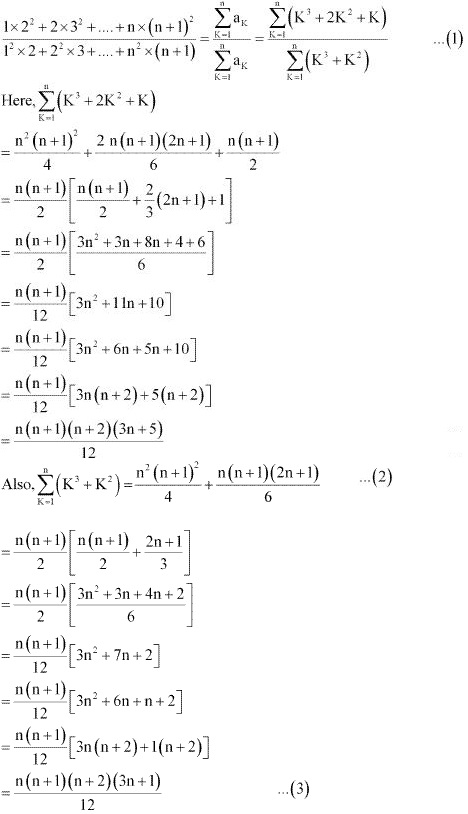From (1), (2), and (3), we obtain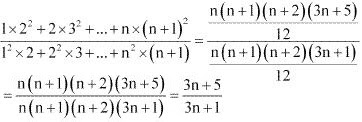Thus, the given result is proved.

27. A farmer buys a used tractor for Rs 12000. He pays Rs 6000 cash and agrees to pay the balance in annual installments of Rs 500 plus 12% interest on the unpaid amount. How much will be the tractor cost him?

Solution

It is given that the farmer pays Rs 6000 in cash.
Therefore, unpaid amount = Rs 12000 – Rs 6000 = Rs 6000
According to the given condition, the interest paid annually is
12% of 6000, 12% of 5500, 12% of 5000, …, 12% of 500
Thus, total interest to be paid = 12% of 6000 + 12% of 5500 + 12% of 5000 + … + 12% of 500
= 12% of (6000 + 5500 + 5000 + … + 500)
= 12% of (500 + 1000 + 1500 + … + 6000)
Now, the series 500, 1000, 1500 … 6000 is an A.P. with both the first term and common difference equal to 500.
Let the number of terms of the A.P. be n.
∴ 6000 = 500 + (n – 1) 500
⇒ 1 + (n – 1) = 12
⇒ n = 12
∴Sum of the A.P = (12/2) [2(500) + (12 -1)(500)] = 6[1000 + 5500] = 6(6500) = 39000
Thus, total interest to be paid = 12% of (500 + 1000 + 1500 + ... + 6000)
= 12% of 39000 = Rs 4680
Thus, cost of tractor = (Rs 12000 + Rs 4680) = Rs 16680

28. Shamshad Ali buys a scooter for Rs 22000. He pays Rs 4000 cash and agrees to pay the balance in annual installment of Rs 1000 plus 10% interest on the unpaid amount. How much will the scooter cost him?

Solution

It is given that Shamshad Ali buys a scooter for Rs 22000 and pays Rs 4000 in cash.
∴Unpaid amount = Rs 22000 – Rs 4000 = Rs 18000
According to the given condition, the interest paid annually is
10% of 18000, 10% of 17000, 10% of 16000 … 10% of 1000
Thus, total interest to be paid = 10% of 18000 + 10% of 17000 + 10% of 16000 + … + 10% of 1000
= 10% of (18000 + 17000 + 16000 + … + 1000)
= 10% of (1000 + 2000 + 3000 + … + 18000)
Here, 1000, 2000, 3000 … 18000 forms an A.P. with first term and common difference both equal to 1000.
Let the number of terms be n.
∴ 18000 = 1000 + (n – 1) (1000)
⇒ n = 18
∴ 1000 + 2000 + .... + 18000 = 18/2 [2(1000) + (18 - 1)1000]
= 9[2000 + 17000]
= 171000
∴ Total interest paid = 10% of (18000 + 17000 + 16000 + … + 1000)
= 10% of Rs 171000 = Rs 17100
∴Cost of scooter = Rs 22000 + Rs 17100 = Rs 39100

29. A person writes a letter to four of his friends. He asks each one of them to copy the letter and mail to four different persons with instruction that they move the chain similarly. Assuming that the chain is not broken and that it costs 50 paise to mail one letter. Find the amount spent on the postage when 8th set of letter is mailed.

Solution

The numbers of letters mailed forms a G.P.: 4, 42, … 48
First term = 4
Common ratio = 4
Number of terms = 8
It is known that the sum of n terms of a G.P. is given by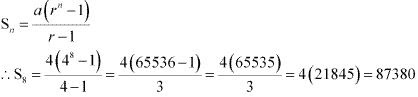It is given that the cost to mail one letter is  50 paisa.
Cost of mailing 87380 letters Rs 87380 × 50/100 = Rs 43690
Thus, the amount spent when 8th set of letter is mailed is Rs 43690.

30. A man deposited Rs 10000 in a bank at the rate of 5% simple interest annually. Find the amount in 15th year since he deposited the amount and also calculate the total amount after 20 years.

Solution

It is given that the man deposited Rs 10000 in a bank at the rate of 5% simple interest annually.
∴ Interest in first year  = (5/100) × Rs 10000 = Rs 500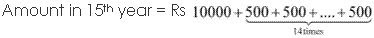= Rs 10000 + 14 × Rs 500
= Rs 10000 + Rs 7000
= Rs 17000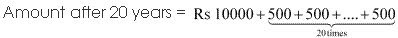= Rs 10000 + 20 × Rs 500
= Rs 10000 + Rs 10000
= Rs 20000

31. A manufacturer reckons that the value of a machine, which costs him Rs 15625, will depreciate each year by 20%. Find the estimated value at the end of 5 years.

Solution

Cost of machine = Rs 15625
Machine depreciates by 20% every year.
Therefore, its value after every year is 80% of the original cost i.e., 4/5 of the original cost.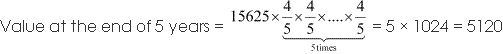Thus, the value of the machine at the end of 5 years is Rs 5120.

32. 150 workers were engaged to finish a job in a certain number of days. 4 workers dropped out on second day, 4 more workers dropped out on third day and so on. It took 8 more days to finish the work. Find the number of days in which the work was completed.

Solution

Let x be the number of days in which 150 workers finish the work.
According to the given information,
150x = 150 + 146 + 142 + …. (x + 8) terms
The series 150 + 146 + 142 + …. (x + 8) terms is an A.P. with first term 150, common difference –4 and number of terms as (x + 8)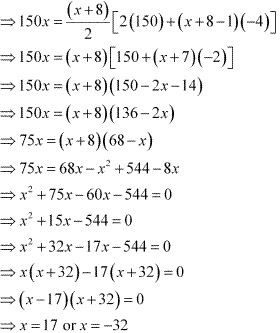However, x cannot be negative.
x = 17
Therefore, originally, the number of days in which the work was completed is  17.
Thus, required number of days = (17 + 8) = 25